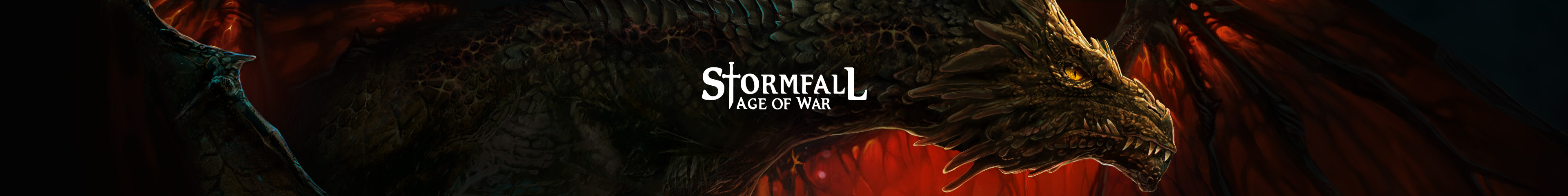All Categories

#SectionsStormfall: Age of War - EN

## Power point presentation by Mehnslayer

Search
Feb 22, 2017, 12:5302/22/17

## Power point presentation by Mehnslayer

Default stats / 40 = units power points (valuable for league quests; train units & kill units. As well as pvp based tournaments and experience)

Pike = 40 Offense / 40 = 1

Archer = 19 Defense / 40 = 0.475

Dwarf = 22 Defense / 40 = 0.55

Paladin = 60 Offense / 40 = 1.5

Arbalester = 30 Defense / 40 = 0.75

Bear rider = 30 Defense / 40 = 0.75

Warden = 60 Defense / 40 = 1.5

Ballista = 60 Defense / 40 = 1.5

Pathfinder = 75 Offense / 40 = 1.875

Huntress = 30 Defense / 40 = 0.75

Nomad = 150 Defense / 40 = 3.75

Knight = 240 Offense / 40 = 6

Barb = 150 Defense / 40 = 3.75

Great lord = 360 Offense / 40 = 9

Reaver = 360 Offense / 40 = 9

Golem = 400 Defense / 40 = 10

Warlock = 720 Offense / 40 = 18

Demon = 415 Defense / 40 = 10.375

Necro = 900 Offense / 40 = 22.5

Griffin = 587.5 Defense / 40 = 14.675

Wyvern = 1440 Offense / 40 = 36

Dragon = 1680 Offense / 40 = 42

Chimera = 900 Offense / 40 = 22.5

Additional section for train units - league quest.

Formula = Resources / PP = PP per resource

Pike = 40 Offense / 40 = 1 -> 300 / 1 = 300 resources per power point

Archer = 19 Defense / 40 = 0.475 -> 120 / 0.475 = 252.63 resources per power point

Dwarf = 22 Defense / 40 = 0.55 -> 192 / 0.55 = 349.09 resources per power point

Paladin = 60 Offense / 40 = 1.5 -> 520 / 1.5 = 346.66 resources per power point

Arbalester = 30 Defense / 40 = 0.75 -> 430 / 0.75 = 573.33 resources per power point

Bear rider = 30 Defense / 40 = 0.75 -> 430 / 0.75 = 573.33 resources per power point

Warden = 60 Defense / 40 = 1.5 -> 860 / 1.5 = 573.33 resources per power point

Ballista = 60 Defense / 40 = 1.5  -> 860 / 1.5 = 573.33 resources per power point

Pathfinder = 75 Offense / 40 = 1.875 -> 1300 / 1.875 = 693.33 resources per power point

Huntress = 30 Defense / 40 = 0.75  -> 520 / 0.75 = 693.33 resources per power point

Nomad = 150 Defense / 40 = 3.75 -> 1760 / 3.75 = 469.33 resources per power point

Knight = 240 Offense / 40 = 6 -> 2166 / 6 = 361 resources per power point

Barb = 150 Defense / 40 = 3.75 -> 1900 / 3.75 = 506.66 resources per power point

Great lord = 360 Offense / 40 = 9 -> 3520 / 9 = 391.11 resources per power point

Reaver = 360 Offense / 40 = 9 -> 5400 / 9 = 600 resources per power point

Golem = 400 Defense / 40 = 10 -> 5000 / 10 = 500 resources per power point

Warlock = 720 Offense / 40 = 18 -> 7823 / 18 = 434.61 resources per power point

Demon = 415 Defense / 40 = 10.375 -> 5300 / 10.375 = 510.84 resources per power point

Necro = 900 Offense / 40 = 22.5 -> 10610 / 22.5 = 471.55 resources per power point

Griffin = 587.5 Defense / 40 = 14.675 -> 7500 / 14.675 = 511.07 resources per power point

Wyvern = 1440 Offense / 40 = 36 -> 19000 / 36 = 527.77 resources per power point

Dragon = 1680 Offense / 40 = 42 -> 21077 / 42 = 501.83 resources per power point

Chimera = 900 Offense / 40 = 22.5 -> 18200 / 22.5 = 808.88 resources per power point

Edit, lower = better... So archer = top tier for power points.Views
11k
3
Apr 25, 2017, 14:0804/25/17
The Power Points per unit are static, regardless of the increased stats of units with Lost Arts and now Unit XP?
Apr 27, 2017, 01:3904/27/17
Apr 27, 2017, 01:39(edited)

cannedam said:

The Power Points per unit are static, regardless of the increased stats of units with Lost Arts and now Unit XP?

Correct, they are static, and also they determine how many experience/pvp/wisdom points you will get on a battle, giving you the same in power point for all the enemy units killed, plus a smaller extra from your units dying. Which is why if you want efficient PvP points, you want to kill non-boosted troops with your boosted units, with an over-whelming victory. Game mechanic that sadly makes the big ones target the small ones for growing.

Alyona KolomiitsevaCommunity Manager
May 3, 2017, 11:4405/03/17
RandomDrop said:

This chart is only 1/2 full in my experience, if you catch an offensive unit stationed in a keep you will only gain what the defense point would grant.
Would you like to alter it or make your own? :) We will gladly support you in that!

•English
•Русский